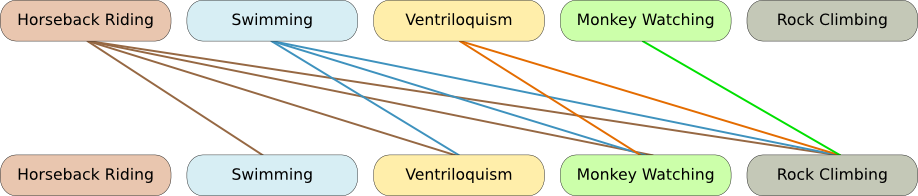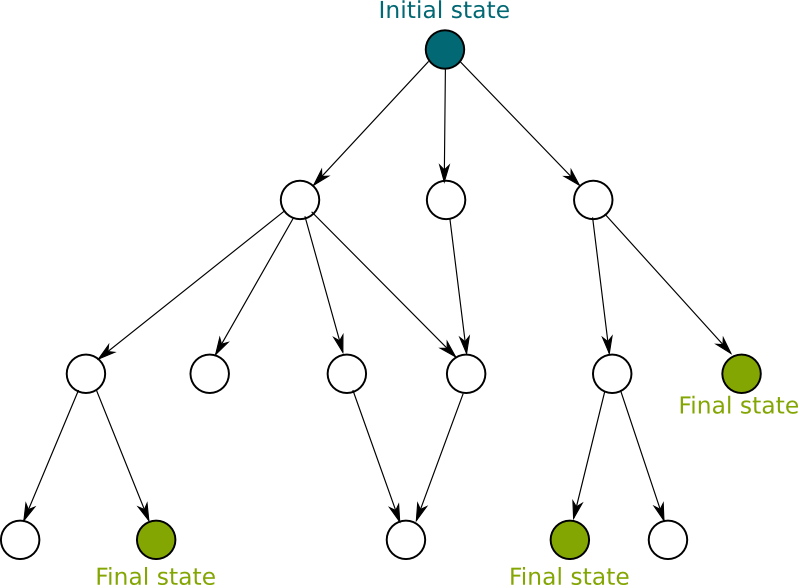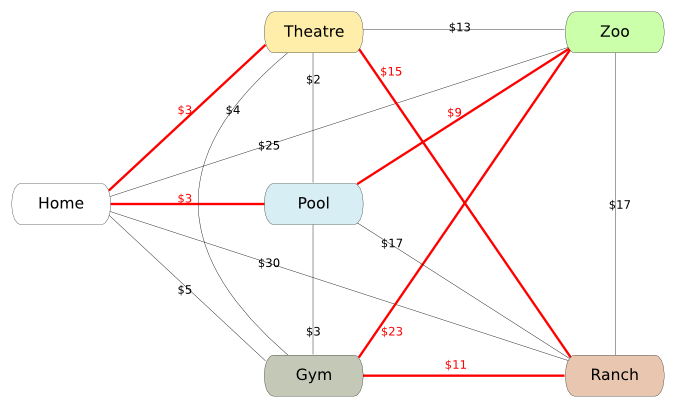# P versus NP: A Crucial Open Problem

The four symbols of the formula $P=NP$ represent the best-known fundamental open problem in computer science and mathematics. First raised by the puzzling Austrian-American mathematician Kurt Gödel, it’s registered among the 7 Millenium Prize problems, and it’s probably the most important open theoretical problem we currently face, because of its many fallouts. In this article, we’ll present P, then NP and then we’ll discuss the open problem. This article deals with a very theoretical problem and I’ll try to make it simple… but it will still be a little bit technical.

In another article, I address another of the millenium prize problems, called the Poincaré conjecture.
It’s going to be technical? Really? Argh…

Humm… I get your point! So, before getting technical, here’s a simplified definition of this crucial problem, which I presented in my talk More Hiking in Modern Math World:

## P

Let’s start by understanding the symbols of the formula. Let’s start with $P$. It represents the set of decision problems that can be solved by a deterministic Turing machine with a polynomial time complexity in the worst case.

What??? What’s a decision problem? What’s a deterministic Turing machine? What’s a polynomial complexity? What do you mean by “worst case”?

Waw, that’s a lot of questions! One at the time, please!

OK! A decision problem is a question that takes into account an input and whose answer is yes or no. For instance, the problem “Are the elements of the array all different?” is a decision problem that takes into account the array as input, and whose answer is either yes or no. Simple, right?

I can’t disagree with that… Now, what’s a deterministic Turing machine?

Before giving you the definition, let’s remark two facts. First, note that deterministic Turing machines are commonly simply called Turing machines. Second, Turing machines correspond to the algorithms we usually define (with tests “if”, loops “while” and “for” and recursions). Although not all algorithms correspond to a Turing machine (and we’ll mention some of those later), most of the algorithms we use or write on computers match the definition of Turing machines.

A Turing machine can be understood as a set of possible states, with transitions between states. At each step of the algorithm, depending on the current state and on the input that’s being read by the machine, a transition towards the next state is taken. Each transition corresponds to a basic operation. For instance, Sheldon’s friendship algorithm (see this video) can be drawn as the following Turing machine, where states are the blue areas and transitions are the arrows:

There are certain specific states. One is the initial state. In Sheldon’s algorithm, the initial state is the one on the top left called “Place Phone Call”. Also, there are states known as final states or accepting states. At theses states, the algorithm finishes. The result of the algorithm is defined by the final state it will eventually reach. In Sheldon’s algorithm, this refers to states “Begin Frienship” and “Partake in Interest”. In decision problems, some states may refer to the answer “true”, others to the answer “false”.

Will transitions always lead to a final state?

No. Look at Sheldon’s friendship algorithm for instance. If it didn’t have a loop count, it might stay in this loop indefinitely. In fact, knowing whether an algorithm will reach a final state is a difficult problem. Indeed, in the late 1930s, Alan Turing, founder of modern computer science, has proved that there does not exist any Turing machine that, given a Turing machine and an input, can determine if the Turing machine will finish with the input. Equivalently, we say that determining whether any Turing machine will halt with any input is undecidable. This problem is known as the halting problem and is a particularly case of Rice’s theorem. This theorem says that non-trivial problems are undecidable. But this is getting complicated and I’m not going to dwell on that.

I don’t really see the link between Turing machines and computers though…

Computers can be modeled by Turing machines. Indeed, each state of the entire memory of the computer can be modeled by a state in the Turing machine. Now, at each step of an algorithm run on a computer, the memory and the input are read, and, based on the given instruction, a decision to overwrite the memory is taken. This leads to a new state of the memory. Thus, the decision to overwrite corresponds to a transition.

Here is a little more on Turing machine. As Alan Turing proved it, there exists a Turing machine, called universal Turing machine, such that, any other Turing machine can be simulated by the universal Turing machine, by writing the instructions on the inputs. For computers, this corresponds to saying that programming languages like C++ or Java are universal Turing machines. You can write instructions, add inputs and give it to those languages to have any algorithm running.

The important fact is that computers can be understood as Turing machines. Thus, algorithms we usually write fall into the definition of P.

I guess I get what Turing machines are… Now, what’s a polynomial time complexity?

Well, for that I first need to talk about the size of the input and the time it takes for an algorithm to finish…

Those two concepts seem quite simple… I mean, the size of the input is the number of bytes, right?

Well, yes, the size of the input is often measured in bytes. But in many case, we prefer to describe the size of the input with a number that is proportional to the size in bytes. For instance, in the case of the array, the size of the input can be considered as the number of elements of the array. Either representation is equivalent to the definitions we’ll see.

And I guess that the time is not measured in seconds…

Well, the problem with measuring the time in seconds is that the time an algorithm will take to finish strongly depend on the power of your processor. And that’s not all, as computers now muli-task everything, if you run an algorithm with the same input twice on the same computer, it will probably not take the same number of seconds to finish. That’s why, when it’s possible, we prefer to measure is the number of basic operations (tests, additions…) done by the computer. This other time is almost proportional to the actual time in seconds, and is equivalent for the definitions we’ll see.

OK, so now you can tell us what polynomial time complexity means…

Yes! A Turing machine has a polynomial time complexity if there exists a polynomial $P$ such that for any input of size $n$, the time it takes for the Turing machine to halt is less than $P(n)$. In this case, we’ll know for sure that the algorithm will finish in a reasonable amount of time for any input. This corresponds to saying that even in the worst case, the complexity time is polynomial in the size of the input.

Some algorithms are known to have a different complexity in average and in the worst case. An algorithm has a polynomial time complexity in average if the means over input of size $n$ of the time the Turing machine takes to halt is less than $P(n)$. However, we need to be careful with such a definition as it’s implied that there is a uniform distribution over input of size $n$. Yet, such a uniform distribution may not model well the reality, or needs to be defined. For instance, the quick sort (see my article on divide and conquer) is known to have a good complexity in average but performs very poorly on already sorted lists, which there are not a lot of if we consider a uniform distribution over mixings of the lists. Yet, in practice, we often work on sorted lists.

I guess you have now all the information to understand what a decision problem that can be solved by a Turing machine with a polynomial time complexity in the worst case is.

I guess… Can you give an example though? Just to be sure I get it…

Sure! Instead of asking the other person to make suggestions one at the time, Sheldon could ask the other person to give a list of suggestions. However, Sheldon is a bit paranoiac, so he’ll make sure that the other person doesn’t mess with him. For instance, he may want to make sure that all suggestions are different from each other.

The problem of testing whether elements of a list are all different is in P. First, it’s a decision problem. Then, let’s consider the following algorithm. We’ll consider every pair of elements of the list. If the two elements of every pair are different from each other, then the answer will be “true”.

How can we list all pairs of the list?

Well, the usual way is to give an order to the elements of the lists. Then, we will consider the matchings of each element with every of its followers in the lists. Here is a graphical representation of that, where each line corresponds to a pairing.This algorithm does answer the question. In addition, if there are $n$ elements in the list, since there are $n(n-1)/2$ pairs of the list, we’ll have to do at most $n(n-1)/2$ iterations, with one basic operation per iteration (the test of whether the former differ from the latter). Thus, the number of operation is less than $P(n) = n(n-1)/2+1$. Thus, the algorithm is polynomial. Thus, this decision problem can be solved by a Turing machine in polynomial time in the worst case: It’s in P.

## NP

Let’s now talk about the two last symbols: NP.

I guess that NP means “Non-Polynomial”, right?

No! Although the “N” does stand for “Non” and the “P” for “Polynomial”, “NP” stands for Non-deterministic Polynomial. It’s the set of decision problems that, if the answer is true, can be solved with a non-deterministic Turing machine in polynomial time in the worst case.

What’s a non-deterministic Turing machine?

A non-deterministic Turing machines is pretty much like a deterministic Turing machine, but has a few differences. Mainly, it enables, given a state and an input, transitions towards several states or none at all. If there is a path using these transitions from the initial state towards a final state, then the machine returns “true”. Because of that, non-deterministic Turing machines are very different from Turing machine. The answer is not read on the final state reached as there may be several final states that can be reached. If an answer is found, it’s necessarily “true”.

As opposed to deterministic Turing machines that can be described as paths from the initial state to a final state via intermediate states using transitions, a non-deterministic Turing machine cannot be drawn as a path. It needs to be consider as an arborescence, that is a structure in which each state has zero, one or several “child” states (even though it’s not exactly an arborescence in the sense of graph theory since there can be several paths from the root to a state, or since there can be cycles). Equivalently, the answer given by the deterministic Turing machine is “true” if there exists a final state in the arborescence. The following figure is a representation of the arborescence of a non-deterministic Turing machine.As you can see, states can have zero, one or several transitions. In this example, since there exists final states in the arborescence, the answer is “true”.

Non-deterministic machines can be understood as machines that do each of the possible transitions simultaneously (by, for instance, branching it to another similar machine) and finishes as soon as one of the path of transitions finds a final state. They can also be understood as lucky guessers that will always choose the right transition, to reach as quickly as possible a final state.

For inputs for which the answer is “true”, we can define the time complexity as the number of transitions of the shortest path towards a final state. Similarly to P, we can define the set of problems such that there exists an non-deterministic Turing machine that correctly answers true with a time complexity that’s bounded by a polynomial function of the size of the input. Such problems are called non-deterministic polynomial, that is they are in NP.

Waw… This is getting complicated…

Fortunately, there exists interesting links between non-deterministic and deterministic Turing machines that can help us deal with NP problems. For one thing, any problem that can be solved with non-deterministic Turing machines can be solved with a Turing machine. In addition, any problem in P is also in NP.

But that’s not all! The most important result is the following. A problem is NP if and only if, for any input, there exists a finite (but potentially enormous) set of decision subproblems that are in P, such that the answer of the problem is true whenever the answers of all decision subproblems are true too.

Can you give an example?

Suppose Sheldon does not know what the proposed activities are. He’ll need to go to each of the places to see what they are and how they work. However he only has $50 for taxis. His problem is finding out whether he’ll be able to visit all those places.The previous figure displays the different places and the cost of taxis from each place to another. The red lines are a possible travel for Sheldon. However, the red travel costs 3+15+11+23+9+3=64 dollars. Since Sheldon only has$50, it’s a travel he can’t undertake.

Why is this problem NP?

## More on Science4All

From Divide and Conquer to ParallelizationBy Lê Nguyên Hoang | Updated:2015-12 | Views: 1737
Divide and conquer is a extremely powerful concept that is being used a lot in computer science, and that can also be applied in real life. We present its application to sorting algorithms. Then we'll talk about a major fundamental open mathematical problem, called P=NC.

Probabilistic Algorithms, Probably BetterBy Lê Nguyên Hoang | Updated:2016-02 | Views: 1952
Probabilities have been proven to be a great tool to understand some features of the world, such as what can happen in a dice game. Applied to programming, it has enabled plenty of amazing algorithms. In this article, we discuss its application to the primality test as well as to face detection. We'll also deal with quantum computers, as well as fundamental computer science open problems P=BPP and NP=BQP.

Cryptography and Number TheoryBy Scott McKinney | Updated:2016-01 | Views: 18766
Over 300 years ago, a mathematician named Fermat discovered a subtle property about prime numbers. In the 1970's, three mathematicians at MIT showed that his discovery could be used to formulate a remarkably powerful method for encrypting information to be sent online. The RSA algorithm, as it is known, is used to secure ATM transactions, online business, banking, and even electronic voting. Surprisingly, it's not too difficult to understand, so let's see how it works.